# User:IssaRice/Computability and logic/Diagonalization lemma

The diagonalization lemma, also called the Godel-Carnap fixed point theorem, is a fixed point theorem in logic.

## Rogers's fixed point theorem

Let$f$ be a total computable function. Then there exists an index$e$ such that$\varphi_e \simeq \varphi_{f(e)}$.

(simplified)

Define$d(x) = \varphi_x(x)$ (this is actually slightly wrong, but it brings out the analogy better).

Consider the function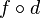$f\circ d$. This is partial recursive, so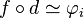$f\circ d \simeq \varphi_i$ for some index$i$.

Now$\varphi_{f(d(i))} \simeq \varphi_{\varphi_i(i)}$ since$f\circ d \simeq \varphi_i$. This is equivalent to$\varphi_{d(i)}$ by definition of$d$. Thus, we may take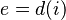$e = d(i)$ to complete the proof.

## Diagonalization lemma

(semantic version)

Let$A$ be a formula with one free variable. Then there exists a sentence$G$ such that$G$ iff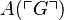$A(\ulcorner G\urcorner)$.

Define$\mathrm{diag}(x)$ to be$\ulcorner C(\ulcorner C\urcorner)\urcorner$ where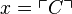$x = \ulcorner C\urcorner$. In other words, given a number$x$, the function$\mathrm{diag}$ finds the formula with that Godel number, then diagonalizes it (i.e. substitutes the Godel number of the formula into the formula itself), then returns the Godel number of the resulting sentence.

Let$B$ be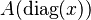$A(\mathrm{diag}(x))$, and let$G$ be$B(\ulcorner B\urcorner)$.

Then$G$ is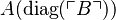$A(\mathrm{diag}(\ulcorner B\urcorner))$, by substituting$x = \ulcorner B\urcorner$ in the definition of$B$.

We also have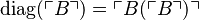$\mathrm{diag}(\ulcorner B\urcorner) = \ulcorner B(\ulcorner B\urcorner)\urcorner$ by definition of$\mathrm{diag}$. By definition of$G$, this is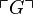$\ulcorner G\urcorner$, so we have$\mathrm{diag}(\ulcorner B\urcorner) = \ulcorner G\urcorner$.

To complete the proof, apply$A$ to both sides of the final equality to obtain$A(\mathrm{diag}(\ulcorner B\urcorner))$ iff$A(\ulcorner G\urcorner)$; this simplifies to$G$ iff$A(\ulcorner G\urcorner)$.

## Comparison table

Some things to notice:

• The two theorems are essentially identical, with identical proofs, as seen by the matching rows. The analogy breaks down slightly at the very end, where we apply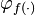$\varphi_{f(\cdot)}$ vs$A(\cdot)$ (the latter corresponds to$f$ until the very end).
• In the partial recursive functions world, it's easy to go from the index (e.g.$e$) to the partial function (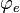$\varphi_e$). In the formulas world it's the reverse, where it's easy to go from a formula (e.g.$A$) to its Godel number$\ulcorner A\urcorner$). I wonder if there is something essential here, or if it is simply some sort of historical accident in notation.
• For the diagonalization lemma, here we have done the semantic version (? I think...), but usually the manipulations are done inside a formal system with reference to some theory$T$ to derive a syntactic result (i.e. we have some theory that is strong enough to do all these manipulations within the object-level language). For partial recursive functions, as far as I know, there is no analogous distinction between semantics vs syntax.
• The diagonalization part is not completely correct/as strong as possible for both proofs. For the partial recursive functions side, we want to make sure that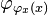$\varphi_{\varphi_x(x)}$ is actually defined in each case. For the logic side, I think often the diagonalization is defined as$\exists x(x = \ulcorner A\urcorner \wedge A)$ so that it is defined for all formulas, not just ones with one free variable. But the essential ideas are all present below, and since this makes the comparison easier, the presentation is simplified.
Step Rogers's fixed point theorem Diagonalization lemma
Theorem statement (note: quantifiers are part of the metalanguage)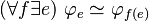$(\forall f \exists e)\ \varphi_e \simeq \varphi_{f(e)}$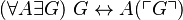$(\forall A \exists G)\ G \leftrightarrow A(\ulcorner G\urcorner)$
Given mapping$f$$A$
Definition of diagonal function$d(x) = \varphi_x(x)$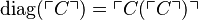$\mathrm{diag}(\ulcorner C\urcorner) = \ulcorner C(\ulcorner C\urcorner)\urcorner$
Composition of given mapping with diagonal function (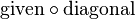$\mathrm{given} \circ \mathrm{diagonal}$)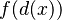$f(d(x))$$A(\mathrm{diag}(x))$
Naming the$\mathrm{given} \circ \mathrm{diagonal}$ composition$f\circ d$ (name not given because compositions are easy to express outside a formal language)$B$
Index of$\mathrm{given} \circ \mathrm{diagonal}$ composition$i$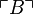$\ulcorner B\urcorner$
Expanding using definition of diagonal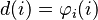$d(i) = \varphi_i(i)$$\mathrm{diag}(\ulcorner B\urcorner) = \ulcorner B(\ulcorner B\urcorner) \urcorner$
The$\mathrm{given} \circ \mathrm{diagonal}$ composition applied to own index (i.e. diagonalization of the composition)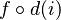$f\circ d(i)$$B(\ulcorner B\urcorner)$
G defined$\varphi_i(i)$ (no equivalent definition)$G$ is$B(\ulcorner B\urcorner)$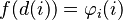$f(d(i)) = \varphi_i(i)$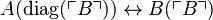$A(\mathrm{diag}(\ulcorner B\urcorner)) \leftrightarrow B(\ulcorner B\urcorner)$
Renaming index$e = d(i)$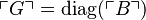$\ulcorner G\urcorner = \mathrm{diag}(\ulcorner B\urcorner)$
Leibniz law to previous row Apply$\varphi_{f(\cdot)}$ to obtain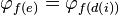$\varphi_{f(e)} = \varphi_{f(d(i))}$ Apply$A(\cdot)$ to obtain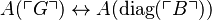$A(\ulcorner G\urcorner) \leftrightarrow A(\mathrm{diag}(\ulcorner B\urcorner))$
Use definition of G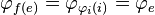$\varphi_{f(e)} = \varphi_{\varphi_i(i)} = \varphi_e$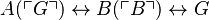$A(\ulcorner G\urcorner) \leftrightarrow B(\ulcorner B\urcorner) \leftrightarrow G$
(Definition of G)?$\varphi_i(i)$ is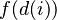$f(d(i))$$G$ is$A(\mathrm{diag}(\ulcorner B\urcorner))$

## Quotes

"All of these theorems tend to strain one's intuition; in fact, many people find them almost paradoxical. The most popular proofs of these theorems only serve to aggravate the situation because they are completely unmotivated, seem to depend upon a low combinatorial trick, and are so barbarically short as to be nearly incapable of rational analysis."

"This is just a lovely result, insightful in its concept and far reaching in its consequences. We’d love to say that the proof was also lovely and enlightening, but to be honest, we don’t have an enlightening sort of proof to show you. Sometimes the best way to describe a proof is that the argument sort of picks you up and shakes you until you agree that it does, in fact, establish what it is supposed to establish. That’s what you get here."

"The brevity of the proof does not make for transparency; it has the aura of a magician’s trick. How did G ̈ odel ever come up with the idea? As a matter of fact, G ̈ odel did not come up with that idea."